# Section G.2. SINGLE COMPLEX FSF FREQUENCY RESPONSE

### G 2 SINGLE COMPLEX FSF FREQUENCY RESPONSE

The frequency response of a single-section complex FSF is Hss(z) evaluated on the unit circle. We start by substituting ejw for z in Hss(z), because z = ejw defines the unit circle. Given an Hss(z) of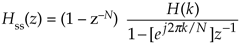we replace the z terms with ejw, giving

Equation G-7Factoring out the half-angled exponentials e–jwN/2 and e–j(w/2 – pk/N), we have

Equation G-8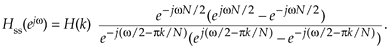Using Euler's identity, 2jsin(a) = eja – e–ja, we arrive at

Equation G-9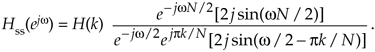Canceling common factors and rearranging terms in preparation for our final form, we have the desired frequency response of a single-section complex FSF:

Equation G-10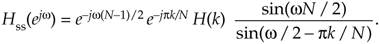Next we derive the maximum amplitude response of a single-section FSF when its pole is on the unit circle and H(k) = 1. Ignoring those phase shift factors (complex exponentials) in Eq. (G-10), the amplitude response of a single-section FSF is

Equation G-11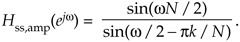We want to know the value of Eq. (G-11) when w = 2pk/N, because that's the value of w at the pole locations, but |Hss(e jw)|w=2pk/N is indeterminate as

Equation G-12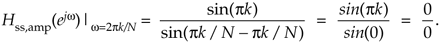Applying the Marquis de L'Hopital's Rule to Eq. (G-11) yields

Equation G-13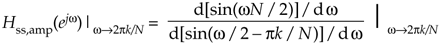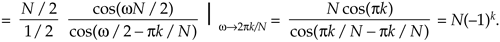The phase factors in Eq. (G-10), when w = 2pk/N, are

Equation G-14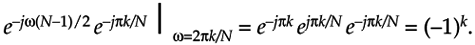Combining the result of Eqs. (G-13) and (G-14) with Eq. (G-10), we have

Equation G-15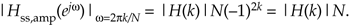So the maximum magnitude response of a single-section complex FSF at resonance is |H(k)|N, independent of k.

URL http://proquest.safaribooksonline.com/0131089897/app07lev1sec2AmazonPrev don't be afraid of buying books Next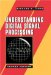Understanding Digital Signal Processing (2nd Edition) ISBN: 0131089897EAN: 2147483647 Year: 2004Pages: 183 Authors: Richard G. Lyons BUY ON AMAZON Flylib.com © 2008-2020. If you may any questions please contact us: flylib@qtcs.net